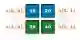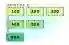# Swift 2D Array Examples

Understand the syntax for 2D arrays. Assign elements and loop over multidimensional arrays.

2D array. On an adventurer's map we see mountains, forests, a lake, a wall. This map is not three-dimensional. The adventurer cannot touch it to see a mountain's height. It is 2D.Array info. With special syntax in Swift, we create multidimensional arrays. We nest brackets—2 or more levels of nesting can be used. An initialization syntax is also available.Array

First example. This program creates a two-dimensional array of Ints. This is an array of arrays—each row can have a different length.
Part 1: We nest levels of brackets to indicate an array of arrays. This example shows the initializer syntax for a 2D array.
Part 2: Here we access 4 individual elements in the points array by specifying 2 separate indexes for each one.Swift program that uses 2D array // Part 1: create a two-dimensional array with nested brackets. let points: [[Int]] = [[10, 20], [30, 40]] // Part 2: access all individual elements. print(points) print(points) print(points) print(points) Output 10 20 30 40

For-loop. Here we see a loop over a 2D array. This is a jagged array, but it uses the same syntax as a rectangular one—they are the same.
Loops: We iterate with a for-loop over all indexes in the arrays. We nest the loops to access all elements.
For
Print: We use print() to write data to the console with trailing newlines. We create a string for each line.
PrintSwift program that uses for-loop, jagged array // Create a constant, jagged array. let units: [[Int]] = [[100, 200, 300], [400, 500], ] // Loop over array and all nested arrays. for var x in 0..<units.count { var line = "" for var y in 0..<units[x].count { line += String(units[x][y]) line += " " } print(line) } Output 100 200 300 400 500 600

String array, append. We can create an empty 2D array and append subarrays to it. This approach is more versatile at creation-time. Here we create a dynamic 2D string array.
Append: We create two empty "row" arrays and append two elements each to them. Then we append those arrays to the "codes" array.
Tip: If you are unsure how large the 2D array needs to be, using logic that appends rows dynamically is a good choice.
Swift program that uses 2D string array, append // An empty 2D string array. var codes = [[String]]() // Create first string array. // ... Append it to the codes 2D array. var row1 = [String]() row1.append("C1") row1.append("A1") codes.append(row1) // Create the second string array row. var row2 = [String]() row2.append("T2") row2.append("S2") codes.append(row2) // Display our 2D string array. print(codes) Output [["C1", "A1"], ["T2", "S2"]]

3D array. Swift supports 3D arrays in the same way as 2D arrays. We nest square brackets in the type. Here we create a three-dimensional array of Ints.
Warning: Often complex arrays like 3D arrays can be rewritten to use dictionaries. This approach can also be more efficient at runtime.
Swift program that creates 3D array // Create a three-dimensional array. // ... The outer array contains two arrays. // ... Those two arrays both contain two arrays. // ... The inner arrays have two values each. let parts: [[[Int]]] = [[[1, 2], [3, 4]], [[5, 6], [7, 8]]] for var a in 0..<parts.count { // Print index of first dimension. print("Outer = \(a)") // Display inner two arrays. for var b in 0..<parts[a].count { var line = "" for var c in 0..<parts[a][b].count { line += String(parts[a][b][c]) } print(line) } print("") } Output Outer = 0 12 34 Outer = 1 56 78

Subscript. Sometimes accessing a 2D array can become confusing or inefficient. A subscript (as part of a class) can validate access to a 2D array with similar calling syntax.
Here: We introduce a WorldMap class. We add a subscript to this class. The WorldMap has a 2D storage array.
Init: We initialize the storage array to be 100 by 100 elements. We do this by appending arrays filled with zeros.
Subscript: This accepts a row and column Int. We call the get and set accessors with two Int arguments (like a method).
Subscript
Finally: We use the WorldMap class and its subscript function. We set values in the storage array and then read them.
Swift program that uses 2D array with subscript in class class WorldMap { var storage = [[Int]]() init() { // Create a 100 by 100 two-dimensional array. // ... Use append calls. for _ in 0..<100 { var subArray = [Int]() for _ in 0..<100 { subArray.append(0) } storage.append(subArray) } } subscript(row: Int, column: Int) -> Int { get { // This could validate arguments. return storage[row][column] } set { // This could also validate. storage[row][column] = newValue } } } // Create our class and use its subscript. // ... This modifies its two-dimensional Int array. var world = WorldMap() world[0, 5] = 100 // Set. world[9, 9] = 120 world[99, 99] = world[0, 5] print(world[0, 0]) // Get. print(world[0, 5]) print(world[9, 9]) print(world[99, 99]) Output 0 100 120 100

Array of dictionaries. An array of dictionaries uses syntax similar to that of a 2D array. We use nested square brackets. A dictionary provides more versatility in lookups.Array, Dictionaries

A summary. Often 2D data structures can be represented in other ways—with dictionaries or sparse arrays. The best (fastest) approach depends on the program and its data.

Home

© 2007-2020 sam allen. send bug reports to info@dotnetperls.com.